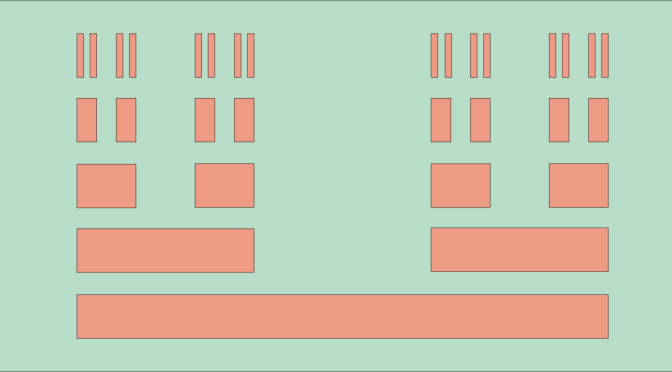Cantor set: a null set having the cardinality of the continuum

Definition of the Cantor set

The Cantor ternary set (named Cantor set below) $$K$$ is a subset of the real segment $$I=[0,1]$$. It is built by induction:

• Starting with $$K_0=I$$
• If $$K_n$$ is a finite disjoint union of segments $$K_n=\cup_k \left[a_k,b_k\right]$$, $K_{n+1}=\bigcup_k \left(\left[a_k,a_k+\frac{b_k-a_k}{3}\right] \cup \left[a_k+2\frac{b_k-a_k}{3},b_k\right]\right)$

And finally $$K=\displaystyle \bigcap_{n \in \mathbb{N}} K_n$$. The Cantor set is created by repeatedly deleting the open middle third of a set of line segments starting with the segment $$I$$.

The Cantor set is a closed set as it is an intersection of closed sets.

The Cantor set has measure $$0$$

$$K_n$$ is the disjoint union of $$2^n$$ closed intervals of length $$3^{-n}$$. As $$K \subset K_n$$, we have $$\mu(K) \le \mu(K_n)=(\frac{2}{3})^n$$ for all $$n \in \mathbb{N}$$ where $$\mu(A)$$ denotes the Lebesgue measure of a measurable subset $$A \subset \mathbb{R}$$. Therefore $$\mu(K)=0$$, i.e. $$K$$ is a null set.

The Cantor set has the cardinality of the continuum

By induction, one can prove that for all $$n \in \mathbb{N}$$:
$K_n = \bigcup_{(\epsilon_1, \dots, \epsilon_n) \in \{0,2\}^n} \left[\sum_{k=1}^n \frac{\epsilon_k}{3^k},\frac{1}{3^n}+\sum_{k=1}^n \frac{\epsilon_k}{3^k}\right]$
Let’s pick-up $$x \in [0,1]$$ and consider its ternary representation $$\displaystyle x = \sum_{k=1}^\infty \alpha_k 3^{-k}$$. For $$(\epsilon_1, \dots, \epsilon_n) \in \{0,2\}^n$$ we have the equivalence:
$\sum_{k=1}^n \frac{\epsilon_k}{3^k} \le x < \frac{1}{3^n}+\sum_{k=1}^n \frac{\epsilon_k}{3^k} \Leftrightarrow \forall k \in \{1, \dots, n\}, \alpha_k = \epsilon_k$ In the case that $$x$$ can be written $$x=\frac{1}{3^n}+\sum_{k=1}^n \frac{\epsilon_k}{3^k}$$, we also have $$x = \sum_{k=1}^\infty \epsilon_k 3^{-k}$$ with $$\epsilon_k = 2$$ for all $$k \ge n+1$$. Finally, $$x \in K$$ if and only if there exist a sequence $$(\epsilon_k) \in \{0,2\}^{\mathbb{N}^*}$$ such that $$x = \sum_{k=1}^\infty \epsilon_k 3^{-k}$$. In other words, $$x$$ belongs to $$K$$ if and only if its ternary representation (proper or improper) contains only the figures $$0$$ and $$2$$. To conclude on the cardinality of $$K$$, we consider the function: $\varphi : \left| \begin{array}{ll} [0,1) & \longrightarrow K\\ x=\sum_{k=1}^\infty \frac{\epsilon_k}{2^k} & \longmapsto \sum_{k=1}^\infty \frac{2 \epsilon_k}{3^k} \end{array} \right.$ $$\varphi$$ is one-to-one. As $$[0,1)$$ has the cardinality of the continuum $$\mathfrak{c}$$, $$K$$ also has the cardinality of the continuum.